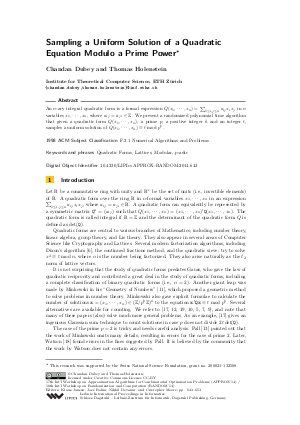Document# Sampling a Uniform Solution of a Quadratic Equation Modulo a Prime Power

### Authors Chandan Dubey, Thomas Holenstein## File

LIPIcs.APPROX-RANDOM.2014.643.pdf
• Filesize: 497 kB
• 11 pages

## Cite As

Chandan Dubey and Thomas Holenstein. Sampling a Uniform Solution of a Quadratic Equation Modulo a Prime Power. In Approximation, Randomization, and Combinatorial Optimization. Algorithms and Techniques (APPROX/RANDOM 2014). Leibniz International Proceedings in Informatics (LIPIcs), Volume 28, pp. 643-653, Schloss Dagstuhl - Leibniz-Zentrum für Informatik (2014)
https://doi.org/10.4230/LIPIcs.APPROX-RANDOM.2014.643

## Abstract

Let p be a prime and k, t be positive integers. Given a quadratic equation Q(x1,x2,...,xn)=t mod p^k in n-variables; we present a polynomial time Las-Vegas algorithm that samples a uniformly random solution of the quadratic equation.
• Lattices
• Modular

## Metrics

• Access Statistics
• Total Accesses (updated on a weekly basis)
0